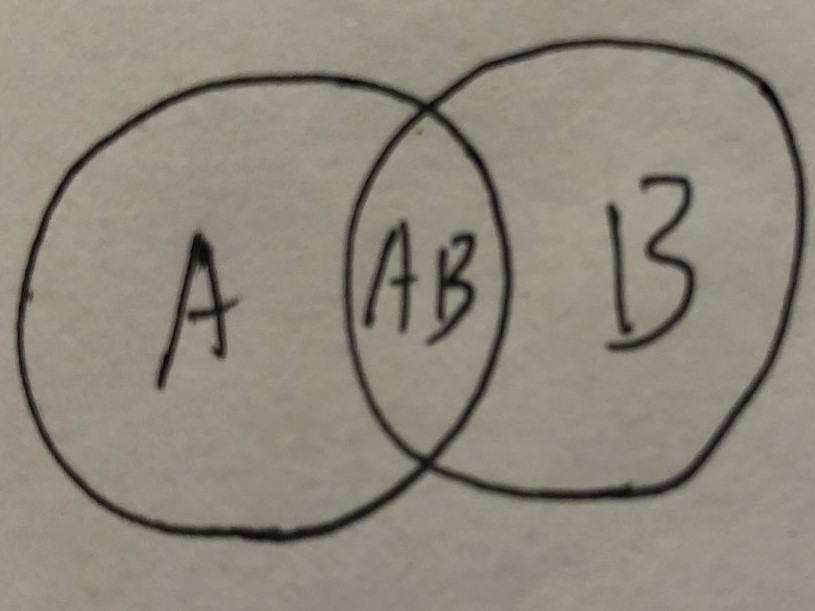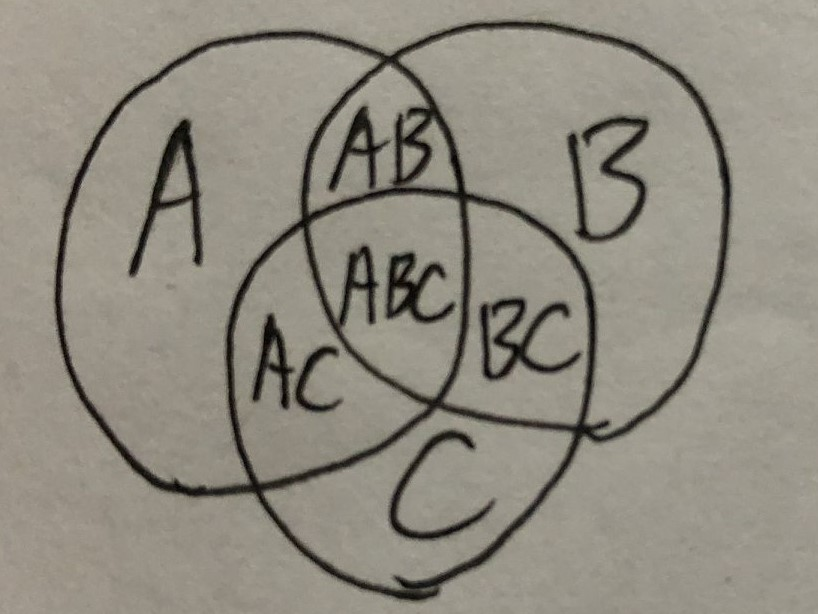• 容斥原理
公式例题求$A$和$B$的总和，很简单，只需要减去一份重复的$AB$
公式： $A+B-AB$求$A$和$B$和$C$的总和
不妨我们以上面的公式想一下，$A$和$B$重叠的时候减去$AB$，剩下$AB$上面那部分就不重复了，即$A+B-AB$，同理，可以对$AC$和$BC$进行同样的操作，那么$AC$和$BC$就都不重复了；而对于中间那部分，$A$和$B$和$C$三个重叠了三次，当我们$A+B-AB$时就减去了一份$ABC$，到最后三份都被减完了，因此我们需要再加一份$ABC$
综上，公式：$A+B+C-AB-AC-BC+ABC$


展开全文算法...